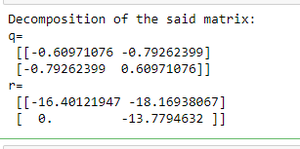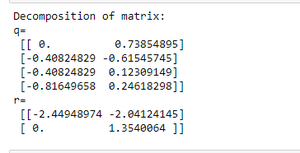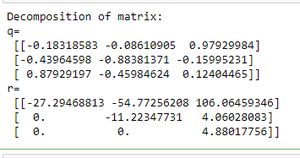Open in App
Not now

# Get the QR factorization of a given NumPy array

• Last Updated : 29 Aug, 2020

In this article, we will discuss QR decomposition or QR factorization of a matrix. QR factorization of a matrix is the decomposition of a matrix say ‘A’ into ‘A=QR’ where Q is orthogonal and R is an upper-triangular matrix. We factorize the matrix using numpy.linalg.qr() function.

Syntax : numpy.linalg.qr(a, mode=’reduced’)

Parameters :

• a : matrix(M,N) which needs to be factored.
• mode : it is optional. It can be :

Below are some examples of how to use the above-described function :

Example 1: QR factorization of 2X2 matrix

## Python3

 `# Import numpy package``import` `numpy as np`` ` `# Create a numpy array ``arr ``=` `np.array([[``10``,``22``],[``13``,``6``]])`` ` `# Find the QR factor of array``q, r ``=`  `np.linalg.qr(arr)`` ` `# Print the result``print``(``"Decomposition of matrix:"``)``print``( ``"q=\n"``, q, ``"\nr=\n"``, r)`

Output :Example 2: QR factorization of 2X4 matrix

## Python3

 `# Import numpy package``import` `numpy as np`` ` `# Create a numpy array ``arr ``=` `np.array([[``0``, ``1``], [``1``, ``0``], [``1``, ``1``], [``2``, ``2``]])`` ` `# Find the QR factor of array``q, r ``=`  `np.linalg.qr(arr)`` ` `# Print the result``print``(``"Decomposition of matrix:"``)``print``( ``"q=\n"``, q, ``"\nr=\n"``, r)`

Output :Example 3: QR factorization of 3X3 matrix

## Python3

 `# Import numpy package``import` `numpy as np`` ` `# Create a numpy array ``arr ``=` `np.array([[``5``, ``11``, ``-``15``], [``12``, ``34``, ``-``51``],``                ``[``-``24``, ``-``43``, ``92``]], dtype``=``np.int32)`` ` `# Find the QR factor of array``q, r ``=` `np.linalg.qr(arr)`` ` `# Print the result``print``(``"Decomposition of matrix:"``)``print``( ``"q=\n"``, q, ``"\nr=\n"``, r)`

Output :My Personal Notes arrow_drop_up